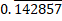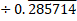# Quiz Discussion

$$\frac{{.009}}{?} = .01$$

Course Name: Quantitative Aptitude

• 1] .0009
• 2] .09
• 3] .9
• 4] 9
##### Solution
No Solution Present Yet

#### Top 5 Similar Quiz - Based On AI&ML

Quiz Recommendation System API Link - https://fresherbell-quiz-api.herokuapp.com/fresherbell_quiz_api

# Quiz
1
Discuss

The price of commodity X increases by 40 paise every year, while the price of commodity Y increases by 15 paise every year. If in 2001, the price of commodity X was Rs. 4.20 and that of Y was Rs. 6.30, in which year commodity X will cost 40 paise more than the commodity Y ?

• 1]

2010

• 2]

2011

• 3]

2012

• 4]

2013

##### Solution
2
Discuss

Given, 168 × 32 = 5376 , then 5.376 ÷ 16.8 is equal to :

• 1] 0.032
• 2] 0.32
• 3] 3.2
• 4] 32
##### Solution
3
Discuss

Express 1999/2111 in decimal :

• 1] 0.893
• 2] 0.904
• 3] 0.946
• 4] 0.986
##### Solution
4
Discuss

The rational number for the recurring decimal 0.125125..... is :

• 1]

63/487

• 2]

119/993

• 3]

125/999

• 4]

None of these

##### Solution
5
Discussis equal to :

• 1]

1/2

• 2]

1/3

• 3]

2

• 4]

10

##### Solution
6
Discuss

Which of the following fractions lies between 2/3 and 3/5 = ?

• 1]

2/5

• 2]

1/3

• 3]

1/15

• 4]

31/50

##### Solution
7
Discuss

617 + 6.017 + 0.617 + 6.0017 = ?

• 1]

62.96357

• 2]

62963.57

• 3]

62.96357

• 4]

629.6357

##### Solution
8
Discuss

The fraction $$101\frac{{27}}{{100000}}$$   in decimal for is

• 1] .01027
• 2] .10127
• 3] 101.00027
• 4] 101.000027
##### Solution
9
Discuss

$$\frac{{\left( {0.1667} \right)\left( {0.8333} \right)\left( {0.3333} \right)}}{{\left( {0.2222} \right)\left( {0.6667} \right)\left( {0.1250} \right)}}$$     is approximately equal to:

• 1] 2
• 2] 2.40
• 3] 2.43
• 4] 2.50
##### Solution
10
Discuss

The expression (11.98 × 11.98 + 11.98 × X + 0.02 × 0.02) will be a perfect square for X equal to:

• 1] 0.02
• 2] 0.2
• 3] 0.04
• 4] 0.4
# Quiz